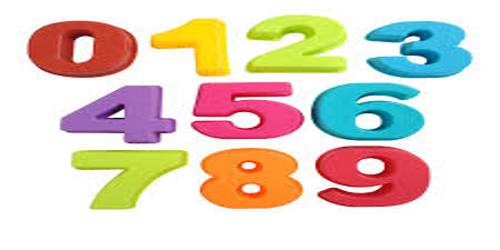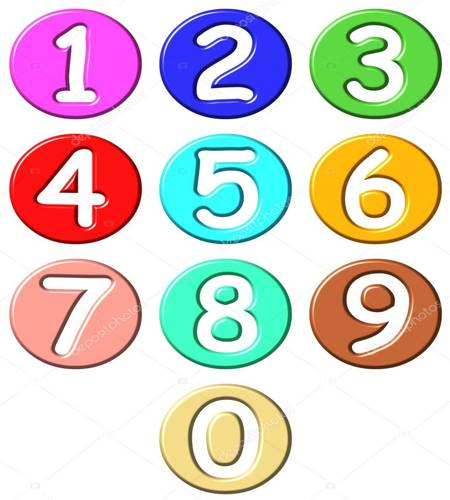Mathematic

# Basic Numbers DefinitionBasic Definitions Relating to Numbers

Basic numbers are the haploid number of chromosomes; specifically the lowest haploid number of a polyploid series.

Some key points of Basic numbers:

• A Number is a quantity.
• A Numeral is a symbol that represents a number.
• Natural Numbers are the normal whole numbers used for counting and ordering.
• A Cardinal Number is a natural number used for counting (e.g. 1, 2, 3 …).
• An Ordinal Number is a natural number used for ordering (e.g. 1st, 2nd, 3rd, …).
• A Whole Number is a number that is not a fractional number.
• Positive Integers are the counting numbers or natural numbers (i.e. 1, 2, 3, …).
• Negative Integers are the whole numbers less than zero (i.e. -1, -2, -3, …).
• Non-negative Integers are the positive integers and zero (i.e. 0, 1, 2, 3, …).
• Integers are the positive and negative whole numbers (i.e. …, -3, -2, -1, 0, 1, 2, 3, …).The basic number systems we use in maths are:

Natural Numbers: The numbers we use for counting are called natural numbers. The set of all natural numbers is denoted by “N”. Hence N= {1,2,3,4……..}.

Whole Numbers: When all the natural numbers and zero are put together, we get a new set of whole numbers. The set of whole numbers is denoted by “W”. Hence W={0,1,2,3,4………}.

Integers: The set containing positive numbers(1,2,3….), negative numbers(-1,-2,-3,…..) together with zero is called as set of integers. We denote the set of integers with “Z”. Hence Z={……-3,-2,-1,0,1,2,3,……..}.

Prime Numbers: Numbers which do not have any other factors except 1 and itself are called prime numbers. Examples: {1,2,3,5,7,11,13,17,19,23,29,31,37,41……….}

Information Sources:

• AAA Math
• My Maths